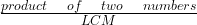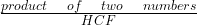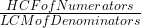##Study notes on HCF and LCM for DSSSB Exam

Here we are providing you Study notes on HCF and LCM which will be helpful for Upcoming CTET and DSSSB Exams

### Important Formula for HCF & LCM:

1.  The product of two numbers = HCF *  LCM.
2.  HCF of given numbers always divides LCM.
3.  HCF =$\frac { product\quad of\quad two\quad numbers }{ LCM }$
4.  LCM =$\frac { product\quad of\quad two\quad numbers }{ HCF }$
5. If d is the h.c.f. of two positive integer a and b, then there exist unique integer m and n, such that d=am+bn.
6. Largest number which divides x,y,z to leave same remainder = HCF of y-x, z-y, z-x.
7. Largest number which divides x,y,z to leave remainder R (i.e. same) = HCF of x-R, y-R, z-R.
8. Largest number which divides x,y,z to leave same remainder a,b,c  = HCF of x-a, y-b, z-c.
9. Least number which when divided by x,y,z and leaves a remainder R in each case = ( LCM of x,y,z) + R.
10. HCF & LCM of fractions
11. HCF of fraction =$\frac { HCF of Numerators }{ LCM of Denominators }$
LCM of fraction =LCM of NumeratorsHCF of Denominators

## Quant Quiz on HCF and LCM for DSSSB Exam

Thanks,

Team Clear IBPSWant to Know More
Please fill in the details below:

Name

ltr
item
Clear CTET - Coaching Institute for CTET, DSSSB and KVS: Study notes on HCF and LCM for DSSSB Exam
Study notes on HCF and LCM for DSSSB Exam
Read the Study notes on HCF and LCM for DSSSB Exam
https://3.bp.blogspot.com/-S7_F9mTKfP0/WdXVJxmk-SI/AAAAAAAAAoY/NNpdTvv0gloiZ9LszvKFrxbXsyz394ZzQCLcBGAs/s320/Study%2Bnotes%2Bon%2Bdsssb.png
https://3.bp.blogspot.com/-S7_F9mTKfP0/WdXVJxmk-SI/AAAAAAAAAoY/NNpdTvv0gloiZ9LszvKFrxbXsyz394ZzQCLcBGAs/s72-c/Study%2Bnotes%2Bon%2Bdsssb.png
Clear CTET - Coaching Institute for CTET, DSSSB and KVS
https://www.clearctet.com/2017/10/study-notes-on-hcf-and-lcm-for-dsssb.html
https://www.clearctet.com/
https://www.clearctet.com/
https://www.clearctet.com/2017/10/study-notes-on-hcf-and-lcm-for-dsssb.html
true
5140436504468951946
UTF-8